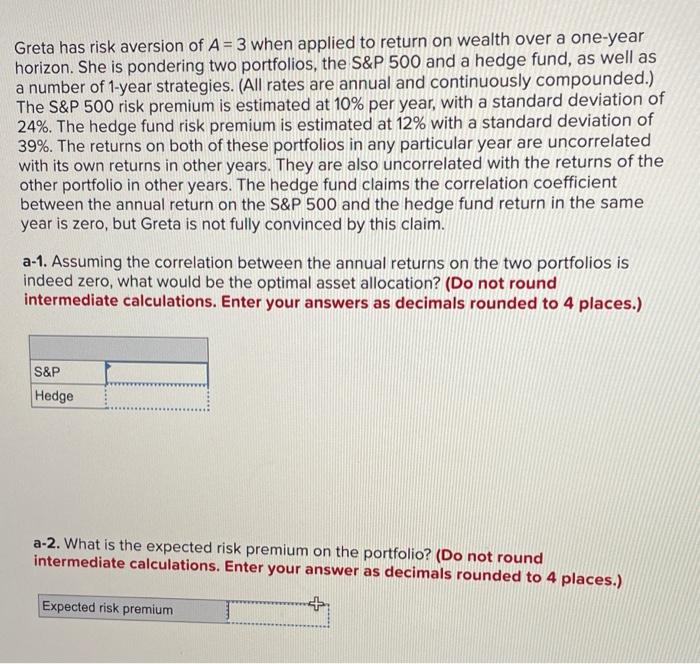# (Solved): please answer correctly. it was previously answered wrong.  Greta has risk aversion of \( A=3 \ ...Greta has risk aversion of \( A=3 \) when applied to return on wealth over a one-year horizon. She is pondering two portfolios, the S\&P 500 and a hedge fund, as well as a number of 1-year strategies. (All rates are annual and continuously compounded.) The S\&P 500 risk premium is estimated at \( 10 \% \) per year, with a standard deviation of \( 24 \% \). The hedge fund risk premium is estimated at \( 12 \% \) with a standard deviation of \( 39 \% \). The returns on both of these portfolios in any particular year are uncorrelated with its own returns in other years. They are also uncorrelated with the returns of the other portfolio in other years. The hedge fund claims the correlation coefficient between the annual return on the S\&P 500 and the hedge fund return in the same year is zero, but Greta is not fully convinced by this claim. a-1. Assuming the correlation between the annual returns on the two portfolios is indeed zero, what would be the optimal asset allocation? (Do not round intermediate calculations. Enter your answers as decimals rounded to 4 places.) a-2. What is the expected risk premium on the portfolio? (Do not round intermediate calculations. Enter your answer as decimals rounded to 4 places.)

We have an Answer from Expert